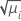# What is a link function?

Generalized linear models include a link function that relates the expected value of the response to the linear predictors in the model. A link function transforms the probabilities of the levels of a categorical response variable to a continuous scale that is unbounded. Once the transformation is complete, the relationship between the predictors and the response can be modeled with linear regression. For example, a binary response variable can have two unique values. Conversion of these values to probabilities makes the response variable range from 0 to 1. When you apply an appropriate link function to the probabilities, the numbers that result range from −∞ to +∞.

The general form of the link function follows:

g(μi) = Xi'β

Minitab provides several link functions which allow you to fit a wide variety of response models. You want to choose a link function that fits your data well. You can use goodness-of-fit statistics to compare models that use different link functions. Certain link functions may be used for historical reasons or because they have special meaning in a discipline. For example, an advantage of the logit link function is that it provides an estimate of the odds ratios. Another example is that the normit link function assumes that there is an underlying variable that follows a normal distribution that is classified into categories.

Minitab offers different link functions for different types of response variables.

Poisson square root